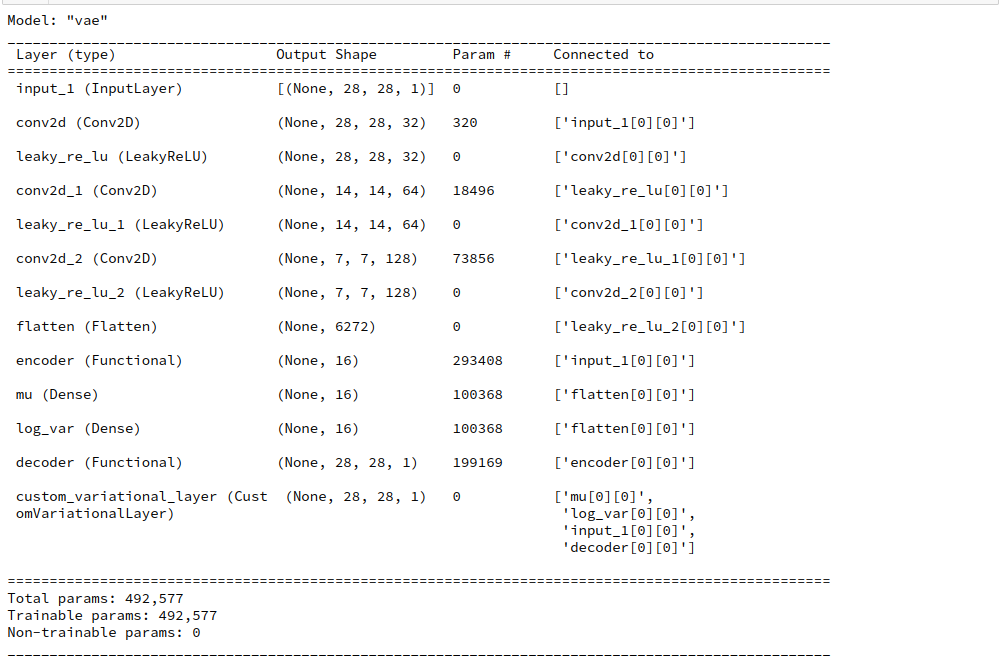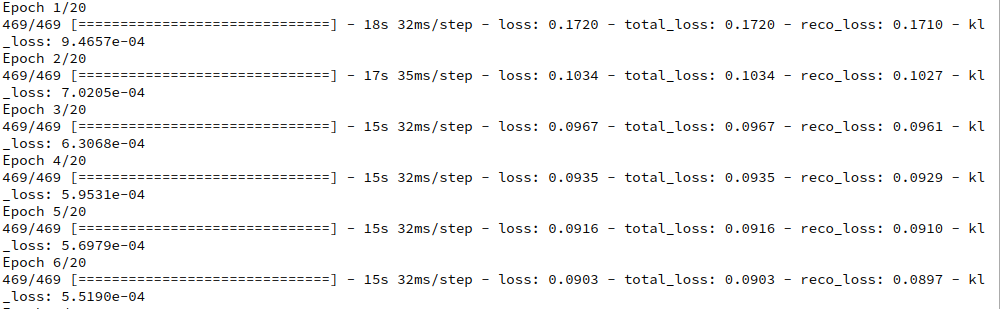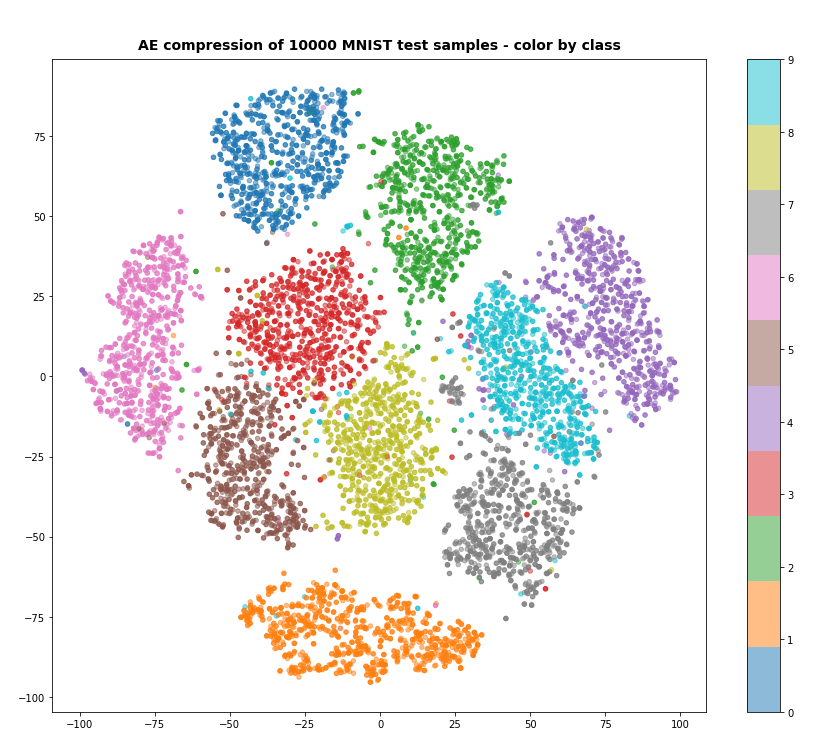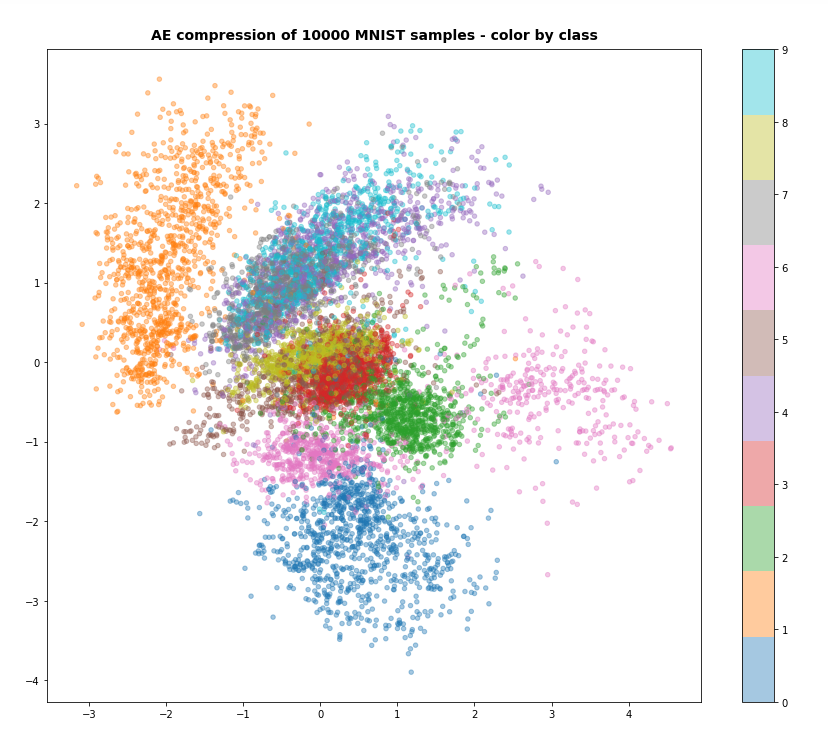# Variational Autoencoder with Tensorflow – IV – simple rules to avoid problems with eager execution

In the last posts of this series

we have seen that it is a bit more difficult to set up a Variational Autoencoder [VAE] with Keras and Tensorflow 2.8 than a pure Autoencoder [AE]. One of the reasons is that we need to include extra layers for a statistical variation of z-points around mean values “mu” with a variance “var” for each sample. In addition a special loss – the Kullback Leibler loss – must be taken into account besides a binary-crossentropy loss to optimize the “mu” and “log_var” values in parallel to a good reconstruction ability of the Decoder.

In the last post we also saw that a too conservative handling of the Kullback-Leibler divergence may lead to problems with the “eager execution mode” of present Tensorflow 2 versions.

In this post I shall first show you how to remedy the specific problem presented in the last post. Sometimes solutions are easy to achieve … :-). But we should also understand the reason for the problem. Some basic considerations will help. Afterward we have a brief look at the performance. At last, we summarize our experiences in some simple rules.

# Eager execution instead of a graph

The next statements are according to my present understanding:
When we designed layered structures of ANNs and related operations with TF 1.x and Keras, Tensorflow built a graph as an intermediate product. The graph contained all mathematical operations in a symbolic way – including the calculation of partial derivatives and gradients. The analysis of the graph by TF afterward lead to a defined sequence of real numerical operations. It is clear that the full knowledge of the graph offers the chance for an optimization of the intended operations, e.g. for ANN-training and error back propagation based on gradient components (=partial derivatives with respect to trainable variables of an ANN, mostly weights). Potential disadvantages of graphs are: Their analysis takes time and it has to be completed before any numerical operations can be started in the background. This in turn means that we cannot test code directly within a sequence of Python statements.

In an eager execution environments planned operations instead are evaluated immediately as the related tensors occur and in case of neural networks as their relation to (weight) variables of interest are properly defined. This includes the calculation of partial derivatives (see my post on error backward calculation for MLPs) with respect to these weights. A requirement is that the operations (= mathematical functions) on specific tensors (represented by matrices) must be well defined. Such operations can be defined by a TF2 math operations directly applied to user defined tensors in a Python statement. But they can also be encapsulated in user or Keras defined functions and combined in complicated ways – provided that it is clear how the chain rule must be applied. As the relation between the trainable variables of neighboring Keras layers in a neural network is well defined also the gradient contributions of two neighbor layers to any loss function is properly defined – and can be calculated already during the forward pass through a neural network. At least in principle we can get resulting tensor values directly or asap during forward propagation wherever possible.

As there are no graphs in eager execution, automatic differentiation based on a graph analysis is not possible without some help. Something has to track operations and functions applied to tensors and record resulting gradient components (i.e. partial derivative values) during a forward pass through a complicated network such that the derivatives can be used during error back-propagation. The tool for this is Gradient.Tape().

A general interface to TF 2.0 like Keras has to incorporate and use Gradient.Tape() internally. While trainable variables like those of a Keras layer can automatically be watched by Gradient.Tape(), specific user defined operations have to be explicitly registered with Gradient.Tape() if you cannot use some Keras model or Keras layer options. However, when you use Keras to define your models gradient related calculations are done directly already during the forward pass through a network. Whilst moving forward through a defined network’s layers gradient contributions (partial derivatives) are evaluated obeying the chain rule across variables of previous layers, of course. The resulting gradient contributions can later be used and properly combined for error backward calculation.

# A remedy to the problem with the failed approach for the KL loss

Just as a reminder: In the last post I introduced a special layer to take care of the KL loss according to a recipe of F. Chollet in his book on Deep Learning of 2017 (see the precise reference at the end of my last post):

Customized Keras layer class:

```class CustVariationalLayer (Layer):

def vae_loss(self, x_inp_img, z_reco_img):
# The references to the layers are resolved outside the function
x = B.flatten(x_inp_img)   # B: tensorflow.keras.backend
z = B.flatten(z_reco_img)

# reconstruction loss per sample
# Note: that this is averaged over all features (e.g.. 784 for MNIST)
reco_loss = tf.keras.metrics.binary_crossentropy(x, z)

# KL loss per sample - we reduce it by a factor of 1.e-3
# to make it comparable to the reco_loss
kln_loss  = -0.5e-4 * B.mean(1 + log_var - B.square(mu) - B.exp(log_var), axis=1)
# mean per batch (axis = 0 is automatically assumed)
return B.mean(reco_loss + kln_loss), B.mean(reco_loss), B.mean(kln_loss)

def call(self, inputs):
inp_img = inputs
out_img = inputs
total_loss, reco_loss, kln_loss = self.vae_loss(inp_img, out_img)
# We add the loss from the layer

return out_img  # not really used in this approach
```

This layer was added on top of the sequence of Encoder and Decoder: Encoder => Decoder => KL_layer.

```enc_output = encoder(encoder_input)
decoder_output = decoder(enc_output)
KL_layer = CustomVariationalLayer()([mu, log_var, encoder_input, decoder_output])
vae = Model(encoder_input, KL_layer, name="vae")
```

# Making it work …

Can we remedy the approach above by some simple means? Yes, we can. I first list the solution’s code, then discuss it:

```# SOLUTION I: Custom Layer for total and KL loss
# ~~~~~~~~~~~~~~~~~~~~~~~~~~~~~~~~~~~~~~~~~~~~~~~
class CustomVariationalLayer (Layer):
def vae_loss(self, mu, log_var, inp_img, out_img):
bce = tf.keras.losses.BinaryCrossentropy()
reco_loss = bce(inp_img, out_img)
kln_loss  = -0.5e-4 * B.mean(1 + log_var - B.square(mu) - B.exp(log_var), axis=1) # mean per sample
return B.mean(reco_loss + kln_loss), B.mean(reco_loss), B.mean(kln_loss) # means per batch

def call(self, inputs):
mu = inputs
log_var = inputs; inp_img = inputs; out_img = inputs
total_loss, reco_loss, kln_loss = self.vae_loss(mu, log_var, inp_img, out_img)
return inputs  # Not used
```

What is the main difference? Answer: We explicitly provided the tensors as input variables of the function vae_loss()!
Why does it help?

Well, TF2 has to prepare and calculate partial derivatives according to the chain rule of differential calculus. What would you yourself want to know on a mathematical level? You would write down any complicated function with further internal operation as a function of well defined arguments! So: We must tell TF2.x explicitly what the variables, namely tensors, of any defined function or operation are to apply the chain rule properly – whatever we do inside the function. When we had graphs this analysis could be done during the analysis of the graph. However, with eager execution we have to know all rules for the affected tensors when they occur and are operated upon. If we operate on tensors via a function, TF2 needs the functions’s arguments to handle the function and following operations properly according to the chain rule. (The tensors themselves at a layer depend, of course, on matrix operations involving trainable parameters, namely weights with respect to a previous layer, and derivatives of activation functions). By the way: The output of the functions must be defined equally well.

In our original approach the function’s input was not defined. It obviously matters with TF2.x!

As a consequence the summary of our VAE model has become longer than in the last post:# What results do we get for z_dim = 16 and z_dim=2?

For our solution we compile and train like follows:

```vae.compile(optimizer=Adam(), loss=None)
n_epochs = 40
batch_size = 128
vae.fit(x=x_train, y=None, shuffle=True,
epochs = n_epochs, batch_size=batch_size)
```

Note that we do not provide any “y” to fit against. The costs are already fully defined by our special customized layer. If we, however, had used the binary_crossentropy loss in the compile statement we would have had to provide predicted tensors; see below.

On a Nvidia 960 GTX the calculation proceeds for some epochs like:After 40 epochs we get with t-SNE well separated clusters for the test-data:More interesting is the result for z_dim = 2, as we expect a more confined usage of the available z-space. And indeed, if we raise the factor in front of the KL loss e.g. to 6.5e-4, we get something likeWith the exception of “6”-digits the samples use the space between -4 < y < 3.5 and -3 < x < 4.5 in z-space. This area is smaller by roughly a factor of 4 (i.e. 2 in each direction) than the space used of a standard Autoencoder (see the 1st post of this series). So, the KL loss shows an effect.

# Performance?

However, our new approach is not as fast as it could be. What can we do to optimize? First we can get rid of the extra function in the layer. We could work directly on the tensors in the call function. A further step would be to focus only on the KL loss. Why not let Keras organize the stuff for binary_crossentropy? But all this would not change our performance much.

The real problem in our case (suggested by the master, F. Chollet, himself in an older book) is an inefficient layer structure: We cannot deal directly with the partial derivatives where the tensors appear – namely in the Encoder. Thereby an otherwise possible sequence of linear algebra operations (matrix operations), which could be optimized for error back propagation, is interrupted in a complicated way at the special layers mu and log_var. So, it appears that a strategy which would encapsulate our KL loss calculation in a specific layer of the Encoder would boost performance. This is indeed the case. I will show the solution in my next post, but give you an idea of the performance gain, already:

Instead of 15 secs as above per epoch we are going to need only 10 to 11 secs.

# What have we learned? Two rules …

I see two basic rules which I personally was not aware of before:

• If you need to perform complex calculations based on layer related tensors to get certain loss contributions and if you want to use the result with pre-defined Keras functions as “layer.add_loss()” and “model.add_loss()” then provide the result tensors explicitly as input variables to the Keras functions. You can use separate personal functions ahead to perform the required tensor operations, but these functions must also have all layer based tensors as explicit input variables and an explicit tensor as output.
• If possible apply your calculations within special layers closely following he layers which provide the tensors your loss contribution depends on. Best before new trainable variables are introduced. Use the special layer’s add_loss() method. Try to verify that your operations fit into a layer related sequence of matrix operations whose values are needed later for error backward propagation, but are calculated already during the forward pass.

The first rule can be symbolized by something like

```# Model definition
...
layer1 = Keras_defined_layer()   #e.g. Dense()
...
layer2 = Keras_defined_layer()   # e.g. Activation()
...
model = Model(....)

# cost calculation
res_tensor_cost_contribution = complex_personal_function( layer1, layer2 )
```

• Try if TF2 math tensor operations are faster than tensorflow.keras.backend operations. I do not think so, but …

# Three strategies to avoid problems with TF 2.8 and VAEs

In the following posts I am going to pursue three ways to handle the KL loss:

1. We add a layer to the Encoder and perform the required KL loss calculation there. We have to take care of a proper output of such a layer not to disrupt the combination of the Encoder with the Decoder. This is in my opinion the most elegant and also the fastest option. It also fits perfectly into the Keras philosophy of defining models via layers. And we can use the Keras compile() and fit() functions seamlessly.
2. We calculate the loss after combining the Encoder and Decoder to a VAE-model – and add the KL loss to our VAE model via its add_loss() method. This is a possible and well defined variant as it separates the loss operations from the VAE’s layer structure. Very similar to what we did above – but probably not the fastest method for VAEs.
3. We use Gradient.Tape() directly to define an individual training step for our Keras based VAE model. This method will prove to be a fast and very flexible method. But in a way it leaves the path of using only Keras layers to define and fit neural network models. Nevertheless: Although it requires a different view on the Keras interface to TF2.x it is certainly the future we should get used to – even if we are no Keras and TF specialists.

# Conclusion

In this post we saw that some old recipes for VAE design with Keras can still be used with some minor modifications. Two rules show us different ways to make Keras based VAE-ANNs work together with TF2.8. In the next post of this series
Variational Autoencoder with Tensorflow – V – a customized Encoder layer for the KL loss
we shall build a VAE with an Encoder layer to deal with the Kullback-Leibler loss.